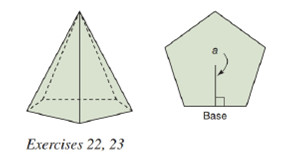Chapter 9.2, Problem 22EElementary Geometry For College St...

7th Edition
Alexander + 2 others
ISBN: 9781337614085

Solutions

Chapter
SectionElementary Geometry For College St...

7th Edition
Alexander + 2 others
ISBN: 9781337614085
Textbook Problem

In a regular pentagonal pyramid, each lateral edge measures 8 i n . , and each base edge measures 6 i n . The apothem of the base measures 4.1 i n . a) Find the lateral area of the pyramid.b) Find the total area of the pyramid.To determine

(a)

To find:

The lateral area of the pyramid.

Explanation

Given:

A regular pentagonal pyramid, each lateral edge measures 8in., and each base edge measures 6in. and the apothem of the base measures 4.1in.

Properties used:

A pyramid is made by connecting a base to an apex. The base is flat with straight edges, no curves, hence, a polygon and all other faces are triangles.

A regular pyramid is a pyramid whose base is a regular polygon and whose lateral edges are all congruent.

In a regular pyramid with slant height l, height h, apothem a, lateral edge e, and radius r,

The slant height l of a regular pyramid is the altitude from the vertex (apex) of the pyramid to the base of any of the congruent lateral faces of the regular pyramid.

The lateral area L of a regular pyramid with slant height of length l and perimeter P of the base is given by

L=12lP.

In a regular pyramid, the lengths of the apothem a of the base, the altitude h, and the slant height l satisfy the Pythagorean Theorem; that is, l2=a2+h2.

Calculation:

From the figure below

Lateral edge measures 8in. i.e. e=8in., and each base edge measures 6in. i.e. perimeter of the pentagonal base is P=5×6=30in.

The apothem of the base measures 4.1in. i.e. a=4.1in.

Calculating OA¯, applying Pythagorean theorem in ΔAOB,

OA¯2=OM¯2+AM¯2OA¯2=(4.1)2+(62)2OA¯2=16

To determine

(b)

To find:

The total area of the pyramid.

Still sussing out bartleby?

Check out a sample textbook solution.

See a sample solution

The Solution to Your Study Problems

Bartleby provides explanations to thousands of textbook problems written by our experts, many with advanced degrees!

Get Started